• 有向图拓扑排序 前言 本文介绍有向图拓扑排序算法的思路及代码实现，首先讲解什么是拓扑排序，其次介绍实现拓扑排序需要的检测有向图是否有环的算法及顶点排序算法，最终实现有向图的拓扑排序。 一、什么是拓扑排序...
有向图拓扑排序
前言
本文介绍有向图拓扑排序算法的思路及代码实现，首先讲解什么是拓扑排序，其次介绍实现拓扑排序需要的检测有向图是否有环的算法及顶点排序算法，最终实现有向图的拓扑排序。
一、什么是拓扑排序？

给定一副有向图，将所有的顶点排序，使得所有的有向边均从排在前面的元素指向排在后面的元素，此时就可以明
确的表示出每个顶点的优先级。如对下图进行拓扑排序：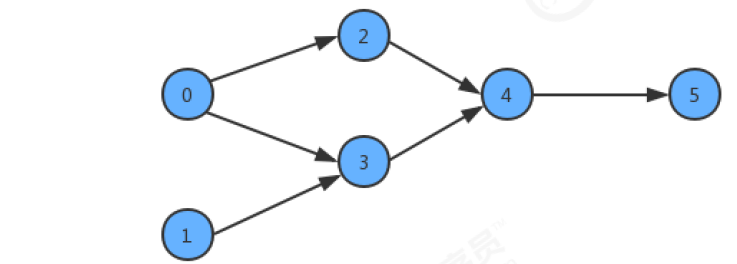拓扑排序结果为：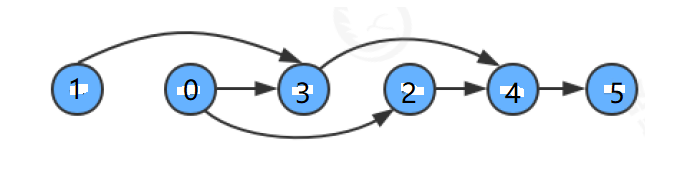根据拓扑排序的概念，如果对有向图进行拓扑排序，那么图中必须没有环，否则，就不能进行拓扑排序，然后在图中无环的情况下，再进行顶点排序，最终实现拓扑排序。

二、检测有向图中是否有环

算法思路：基于深度优先搜索算法检测图中是否有环。1. 定义boolean辅助数组onStack，以栈的思想标识顶点是否在搜索中；2. 在深度优先搜索中，不断检测当前搜索的顶点是否在栈中（即当前顶点的值是否为true，如果为true，则在栈中，否则不在栈中），如果在栈中，说明该顶点被重复搜索到，代表图中有环；3. 每个顶点深度优先搜索完成，onStack需要出栈（即将当前索引对应的值修改为false），为下个顶点搜索做准备
示例：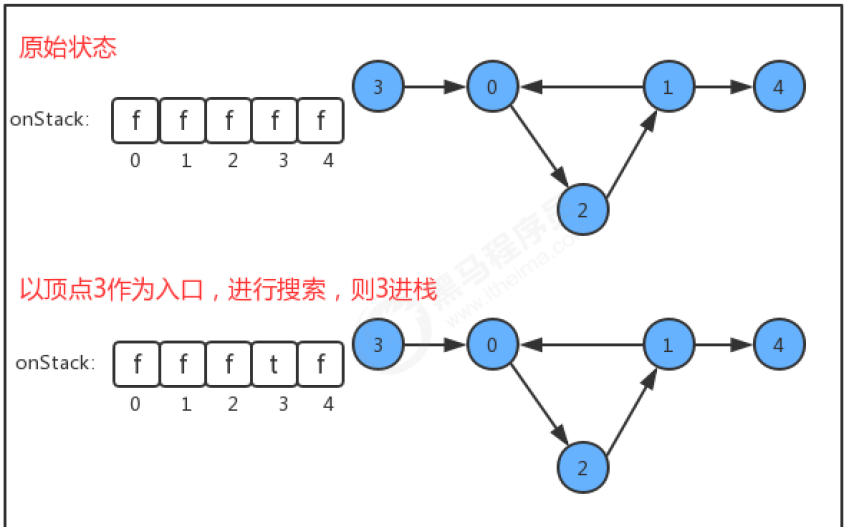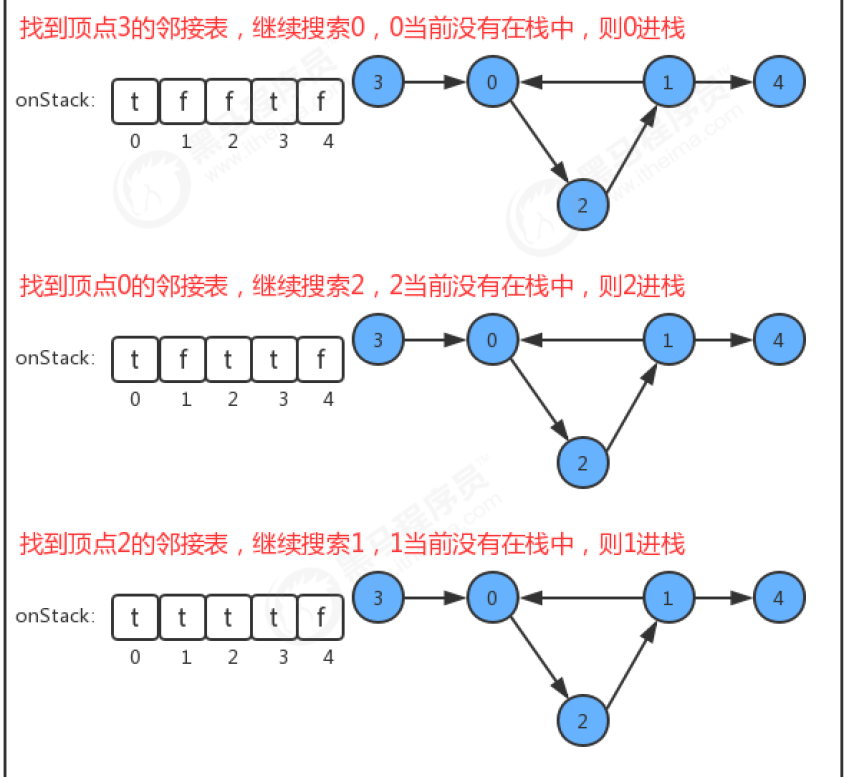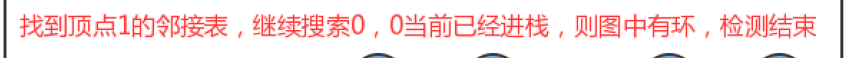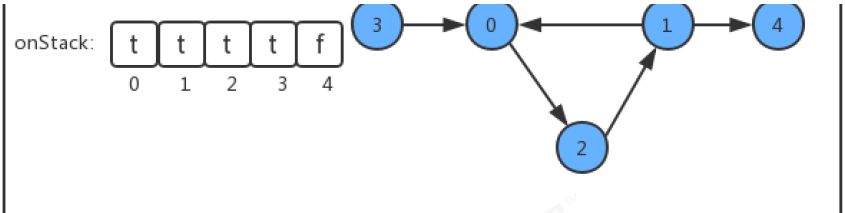代码实现

public class DirectedCycle {
private boolean[] marked;//索引代表顶点，用于标识顶点是否搜索过,是深度优先搜索的复杂数组
private boolean hasCycle;//记录图中是否有环
private boolean[] onStack; //索引代表顶点，使用栈的思想，记录当前顶点有没有已经处于正在搜索的栈上，如果有，则证明有环。

//创建一个检测环对象，检测图G中是否有环
DirectedCycle(DirectGraph G)
{
this.marked=new boolean[G.V()];//用于标识顶点是否搜索过
this.hasCycle=false;
this.onStack=new boolean[G.V()];//用于标识顶点是否在搜索中

//遍历所有顶点，将未搜索过的顶点作为入口，进行深度优先遍历，检测是否有环，一旦检测到有环，则结束；
//因为对于不连通图，有很多个子图，也许某个子图存在环，因此，要对每个子图进行深度优先遍历检测，而不能只检测某一个子图。
for (int v = 0; v < G.V(); v++) {
if (!marked[v])
dfs(G,v);//每次搜索一个子图，判断子图内是否有环，如果没环，继续搜索下一个子图（一次搜索后，未搜索的顶点一定在另一个子图中）
}
}

//基于深度优先搜索，检测图G中是否有环
private void dfs(DirectGraph G,int v)
{
//1.当前顶点标记为已搜索
marked[v]=true;
//2.当前顶点入栈
onStack[v]=true;
//3.递归深度优先遍历，检查遍历结点是否已经在栈中，如果在栈中，则表明该顶点被两次搜索到，证明有环，则结束
for (Integer w : G.adj(v)) {
if (!marked[w])
dfs(G,w);
//如果该顶点已经被搜索过，且如果该顶点在搜索的路径上，则代表又一次搜索到该顶点，证明有环，结束搜索。
if (onStack[w]) {
hasCycle = true;
return;
}
}
//4.当前顶点出栈，为下一个节点作为入口，检测是否有环做准备(为什么需要这样，图2.png可以解释)
onStack[v]=false;
}
//判断当前有向图G中是否有环
public boolean hasCycle()
{
return hasCycle;
}
}

代码中为什么每个顶底深度优先搜索完成后onStack需要出栈，下图可以解释：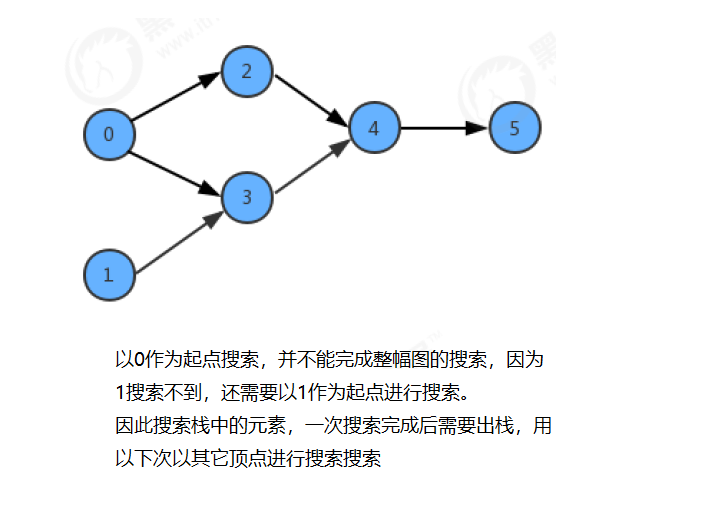三、基于深度优先的顶点排序

拓扑排序使得所有的有向边均从排在前面的元素指向排在后面的元素，要实现这一需要，可以通过顶点排序进行实现。
顶点排序算法思路：1. 定义栈stack用于存储顶点排序的结果；2. 基于深度优先搜索算法，每个顶点深度优先搜索完成后，将该顶点入栈；3. 依次弹出栈中顶点，即为满足拓扑排序要求的顶点序列。
顶点排序示例：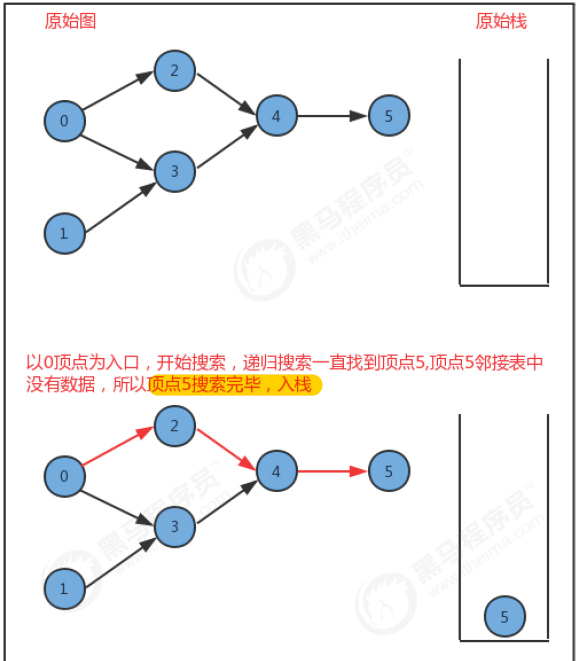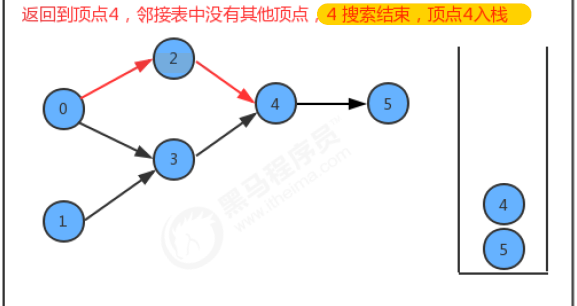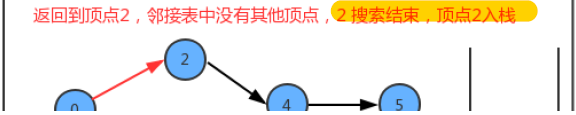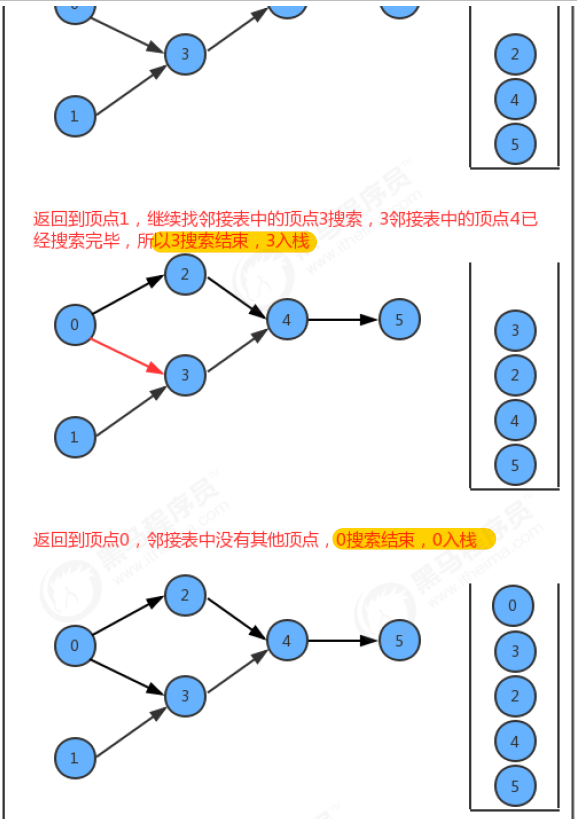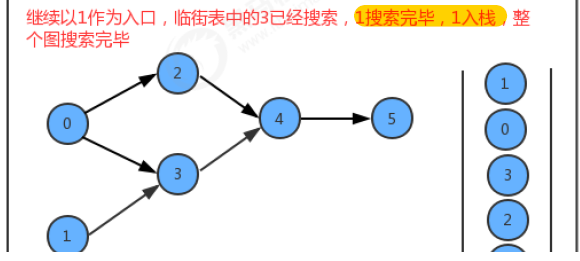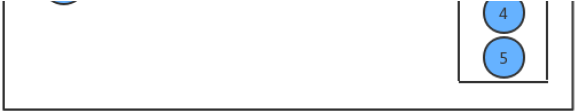代码实现

public class DepthFirstOrder {
private boolean[] marked;//索引代表顶点，值表示当前顶点是否已经被搜索
private Stack<Integer> reversePost;//使用栈，存储顶点序列，打印出栈中的顶点，即是排序后的顶点

public DepthFirstOrder(DirectGraph G)
{
//初始化辅助变量
this.marked=new boolean[G.V()];//默认全部赋值为false
this.reversePost=new Stack<Integer>();
//对每一个未搜索过的顶点进行深度优先遍历
for (int v = 0; v < G.V(); v++) {
if (!marked[v])
dfs(G,v);
}
}

//基于深度优先搜索，生成顶点线性序列
private void dfs(DirectGraph G,int v)
{
//1. 将当前顶点标记为已搜索
marked[v]=true;
//2. 遍历当前顶点的邻接表，对邻接表中未搜索的顶点递归调用深度优先搜索
for (Integer w : G.adj(v)) {
if(!marked[w])
dfs(G,w);
}
//3. 当前顶点v深度优先搜索完毕后，入栈
reversePost.push(v);
}
//获取顶点线性序列
public Stack<Integer> reversePost()
{
return reversePost;
}
}

四、拓扑排序实现

实现了检测是否有环和顶点排序算法，也就完成了拓扑排序，拓扑排序是对上面两个算法的封装。
拓扑排序算法步骤：1. 定义栈用于存储拓扑排序顶底；2. 检测图中是否有环；3. 若有环则不做拓扑排序，若无环则对图进行顶点排序，完成拓扑排序
代码实现

public class TopoLogical {
private Stack<Integer> order; //顶点的拓扑排序

public TopoLogical(DirectGraph G)
{
//1. 检测是否有环
DirectedCycle directedCycle = new DirectedCycle(G);
if (!directedCycle.hasCycle())
{
//2. 调用顶点排序算法
DepthFirstOrder depthFirstOrder = new DepthFirstOrder(G);
this.order=depthFirstOrder.reversePost();
}
}

//判断图G是否有环
public boolean isCycle()
{
return order==null;
}
//获取拓扑排序的所有顶点
public Stack<Integer> order()
{
return order;
}
}



展开全文数据结构
• （1）仅针对有向无环； （2）拓扑排序不唯一，只需保证各节点在上的原始顺序保持不变； （3）实现（可借助辅助队列或栈，时间复杂度为 O(|E|+|V|)）； import java.util.ArrayList; import java.util....
拓扑排序（1）仅针对有向无环图；（2）图的拓扑排序不唯一，只需保证各节点在图上的原始顺序保持不变；（3）实现（可借助辅助队列或栈，时间复杂度为O(|E|+|V|)）；import java.util.ArrayList;
import java.util.LinkedList;
import java.util.List;
import java.util.Queue;

class CycleFoundException extends Exception {}

public class Test {
public static class GrapNode {
public String name;
public int indegree = 0;
public List<GrapNode> neighbors = new ArrayList<GrapNode>();
GrapNode(String name) {
this.name = name;
}
public void addNeighbors(GrapNode... nodes) {
for(GrapNode node : nodes) {
node.indegree++;
neighbors.add(node);
}
}
}
public static List<String> topSort(GrapNode... grap) throws CycleFoundException{
Queue<GrapNode> queue = new LinkedList<GrapNode>();
List<String> sorted = new ArrayList<String>();
for(GrapNode node : grap) {
if(node.indegree == 0)
queue.add(node);
}
while(!queue.isEmpty()) {
GrapNode node = queue.remove();
sorted.add(node.name);
for(GrapNode n : node.neighbors) {
if(--n.indegree == 0)
queue.add(n);
}
}
if(sorted.size() < grap.length)
throw new CycleFoundException();
return sorted;
}
public static void main(String[] args) {
GrapNode v1 = new GrapNode("v1");
GrapNode v2 = new GrapNode("v2");
GrapNode v3 = new GrapNode("v3");
GrapNode v4 = new GrapNode("v4");
GrapNode v5 = new GrapNode("v5");
GrapNode v6 = new GrapNode("v6");
GrapNode v7 = new GrapNode("v7");
v1.addNeighbors(v2,v3,v4);
v2.addNeighbors(v4,v5);
v3.addNeighbors(v6);
v4.addNeighbors(v3,v6,v7);
v5.addNeighbors(v4,v7);
v7.addNeighbors(v6);
try {
List<String> sorted = topSort(v1,v2,v3,v4,v5,v6,v7);
for(String s : sorted) {
System.out.print(s+", ");
}

} catch (CycleFoundException e) {
e.printStackTrace();
}
}
}/*output:
v1, v2, v5, v4, v3, v7, v6,
*///:~
展开全文• PAGE 数据结构课程设计 设计说明书 有向图拓扑排序算法的实现 学生姓名 樊佳佳 学号 1318064017 班级 网络工程1301 成绩 指导教师 申静 数学与计算机科学学院 2016年1月4日 课程设计任务书 20152016学年第一学期 ...
• 文章目录有向图拓扑排序实现思路 有向图 有向图在计算机中有广泛的应用。有向图的顶点之间的联系是描述现实世界的有利工具，如 计算机的任务调度系统，根据多任务之间的先后关联需要给出任务的执行顺序，拓扑排序...
文章目录有向图拓扑排序实现思路Java代码实现封装顶点的类封装用于topo排序的队列的类封装图的类测试图的类
有向图
有向图在计算机中有广泛的应用。有向图的顶点之间的联系是描述现实世界的有利工具，如 计算机的任务调度系统，根据多任务之间的先后关联需要给出任务的执行顺序，拓扑排序就是可以得到这一顺序的算法。
拓扑排序
给定一幅有向图，将所有的顶点排序，使得所有的有向边均从排在前面的元素指向排在后面的元素（或者说明无法做到这一点）。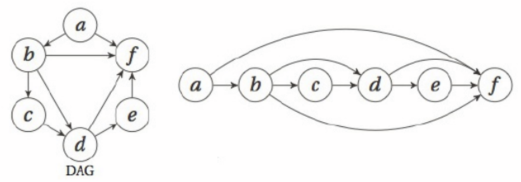注意项：还是以任务调度系统为例，假设一个任务x必须在任务y之前完成，任务y必须在任务z之前完成，任务z又必须在任务x之前完成，这样的问题肯定是无解的。也就是说，拓扑排序能得出结果的前提是图必须是有向无环图（Directed Acyclic Graph，DAG），即一幅不含有环路的有向图。
实现思路

遍历图中所有的顶点，将入度为0的顶点如队列（如果没有这样的顶点，就说明存在环）。
从队列中出一个出一个顶点，打印该顶点，并更新该顶点指向的邻接节点的入度（减1）。如果邻接节点的入度更新之后变为了0，就将邻接节点放入队列。
循环步骤2，直至队列为空。

Java代码实现
封装顶点的类
package graph;

/**
* 顶点的类
*/
public class Vertex {
public char label;
public boolean isVisited;
public int degree;//拓扑排序入度

public Vertex(char label){
this.label = label;
isVisited = false;//默认没有被访问
degree = 0;
}
}


封装用于topo排序的队列的类
package graph;

/**
* 实现广度优先搜索或者topo排序的队列
*/
public class Queue {
private final int SIZE = 20; //final 定义的变量只能在定义的时候初始化一次，以后不能再做初始化操作。不能再被改变。
private int[] queArray;//存放数据的数组
private int front;//队头
private int rear;//队尾

public Queue(){
queArray = new int[SIZE];
front = 0;
rear = -1;
}

public void insert(int j){
if (rear == SIZE-1){
rear = -1;
}
queArray[++rear] = j;
}

public int remove(){
int temp = queArray[front++];
if (front == SIZE){
front = 0;
}
return temp;
}

public boolean isEmpty(){
return (rear+1 == front || front+SIZE-1 == rear);
}

}


封装图的类
package graph;

public class GraphTopo {
//图的基本属性
private Vertex[] vertexList;//保存顶点的数组
private int[][] adjMat;//邻接矩阵
private int nVerts;//图中存在的节点数量
private final int MAX_VERTS = 20;//初始化一个图中的顶点最大的个数
private Queue topoQueue;//用于拓扑排序的队列

//构造方法
public GraphTopo(){
vertexList = new Vertex[MAX_VERTS];
adjMat = new int[MAX_VERTS][MAX_VERTS];
for (int i=0;i<MAX_VERTS;i++){
for (int j=0;j<MAX_VERTS;j++){
adjMat[i][j] = 0;
}
}
nVerts = 0;//初始状态，图内没有节点

topoQueue = new Queue();

}

//向图中插入新的顶点
public void insert(char label){
vertexList[nVerts++] = new Vertex(label);
}

//更新边，设置顶点的连接关系
public void addEdge(int start, int end){
//有向图只更新一个部分
adjMat[start][end] = 1;
}

//打印指定的顶点中的label
public void displayVertex(int v){
System.out.print(vertexList[v].label);
}

/**
* 实现有向图的拓扑排序
*/
public void topo(){
//计算各个顶点的入度
setDegree();
//1.找到图中入度为0的顶点
int[] zeroDegreeNodes = getZeroDegree();
for (int i:zeroDegreeNodes){
if (i==-1){
//表示数组的该位置没有元素
break;
}
//顶点的序号放入队列
topoQueue.insert(i);
}

int popNodeIndex;
int printNum = 0;//记录已经打印的接地那个数
char[] printNode = new char[nVerts];
while (!topoQueue.isEmpty()){
//从队列中出一个出一个顶点，打印该顶点
popNodeIndex = topoQueue.remove();
//            System.out.print(vertexList[popNodeIndex].label + " ");
printNode[printNum++]=vertexList[popNodeIndex].label;
//更新该顶点指向的邻接节点的入度（减1）
for (int i=0;i<nVerts;i++){
if (adjMat[popNodeIndex][i] == 1){
vertexList[i].degree--;
if (vertexList[i].degree == 0){
//如果邻接节点的入度更新之后变为了0，就将邻接节点放入队列。
topoQueue.insert(i);
}
}
}
}
if (printNum < nVerts){
System.out.println("有向图中存在环，无法进行拓扑排序");
}else {
//打印拓扑排序结果
for(char j:printNode){
System.out.print(j+"  ");
}
System.out.println();
}

}

//设置各节点的入度
public void setDegree(){
int i;
for (i=0;i<nVerts;i++){
for (int j=0;j<nVerts;j++){
if (adjMat[i][j] == 1){
vertexList[j].degree++;
}
}
}

}

//找到图中入度为0的顶点
public int[] getZeroDegree(){
int[] zeroDegreeNodes = new int[nVerts];
int zeroNum = 0;
int i;
for (i=0;i<nVerts;i++){
if (vertexList[i].degree == 0){
zeroDegreeNodes[zeroNum++]=i;
}else {
zeroDegreeNodes[zeroNum++]=-1;//表示该位置没有数据
}
}
return zeroDegreeNodes;
}

}


测试图的类
package graph;

public class GraphMain {
public static void main(String[] args) {
GraphTopo graph = new GraphTopo();

graph.insert('A');//0
graph.insert('B');//1
graph.insert('C');//2
graph.insert('D');//3
graph.insert('E');//4
graph.insert('F');//5

graph.addEdge(0,1);
graph.addEdge(1,2);
graph.addEdge(2,3);
graph.addEdge(3,4);
graph.addEdge(4,5);
graph.addEdge(0,5);
graph.addEdge(1,5);
graph.addEdge(1,3);
graph.addEdge(3,5);

//        graph.insert('0');
//        graph.insert('1');
//        graph.insert('2');
//        graph.insert('3');
//        graph.insert('4');
//        graph.insert('5');
//
//        graph.addEdge(0,2);
//        graph.addEdge(0,3);
//        graph.addEdge(1,3);
//        graph.addEdge(2,4);
//        graph.addEdge(3,4);
//        graph.addEdge(4,5);

graph.topo();

}
}



展开全文• 现在你总共有 n 门课需要选，记为 0 到 n-1。...解题思路：这是是个有向图拓扑排序问题。如果图中存在环，那么就不能产生结果，需要返回空。 利用一个map来保存图，需要先学习的课程为出发点； .

现在你总共有 n 门课需要选，记为 0 到 n-1。
在选修某些课程之前需要一些先修课程。 例如，想要学习课程 0 ，你需要先完成课程 1 ，我们用一个匹配来表示他们: [0,1]
给定课程总量以及它们的先决条件，返回你为了学完所有课程所安排的学习顺序。
可能会有多个正确的顺序，你只要返回一种就可以了。如果不可能完成所有课程，返回一个空数组。
leetcode

是这道题的改进版本。链接
解题思路：这是是个有向图的拓扑排序问题。如果图中存在环，那么就不能产生结果，需要返回空。

利用一个map来保存图，需要先学习的课程为出发点；
深搜每个节点，一条路中，最后搜到的点需要放在最后，这里用一个索引，初始值是数组索引的最大值，搜到一个节点之后，索引递减。因为每次一条路上搜到最后的节点，肯定需要放在更后面的位置。
如果搜到了之前存在的节点，那么就将环标记置位。

class Solution {
Map<Integer, List<Integer>> map = new HashMap<>();
int[] res;
int cnt;
public int[] findOrder(int numCourses, int[][] prerequisites) {
int[] st = new int[numCourses];
res = new int[numCourses];
cnt = numCourses - 1;

for(int i = 0; i < numCourses; i++) map.put(i, new ArrayList<>());

// 将图放到哈希表中，方便遍历
for(int[] p : prerequisites) map.get(p).add(p);

// 分别从各个节点来遍历图
for(int i = 0; i < numCourses; i++) {
// 如果在之前的路径中搜过这个点，那么就不再搜
if(st[i] != 0) continue;
if(!dfs(i, st)) return new int;
}

return res;
}

public boolean dfs(int cur, int[] st) {
// 标记过该点，说明图中有环
if(st[cur] == 1) return false;
// 遍历过该点，说明图中无环
if(st[cur] == -1) return true;
// 标记该店
st[cur] = 1;
for(int i = 0; i < map.get(cur).size(); i++) {
// 再从该点开始遍历
if(!dfs(map.get(cur).get(i), st)) return false;
}

// 说明该点不在环中，给该点状态置为无环，用于剪枝
st[cur] = -1;
// 将当前点的路径写入
res[cnt --] = cur;
return true;

}
}




展开全文算法 dfs java leetcode
• 在没有环的情况下，将上排序为下的样子，因此需要两个基础类，一个是判断是否有有环，一个是顶点排序，前面都记录，这里只是将代码整合一下，整合到拓扑排序的类中。 这里需要注意的是，即使真的环，也能...
• #include&lt;bits/stdc++.h&gt; using namespace std; const int maxn = 1000 + 5; int a[maxn][maxn];//保存两边之间的关系 int c[maxn]; int topo[maxn]; int n, m, t; bool dfs(int u){ ... v...
• * C++: 无回路有向图(Directed Acyclic Graph)的拓扑排序 * 该DAG图是通过邻接表实现的。 * * @author judyge * @date 2014/04/22 */ #include #include #include #include using namespace std; #...
• ## C#有向图拓扑排序

千次阅读 2010-01-23 11:04:00
///  /// 的节点类  ///  public class GraphNode  {  private int inDegree;  private int outDegree;  private string nodeName;  ///  /// 节点入度c# string class null 算法
• 有向图拓扑排序，能够获得访问到某一节点的提前条件。 拓扑排序时不可以实现环和图的拓扑排序。   写一下拓扑排序的实现： 是在联通矩阵上实现的，拓扑排序的算法是： 1.查看连通矩阵是否还有剩余节点...java
• //判断有向图中是否有环 public: void dfs(int u) { visited[u] = 1;//搜索中 for (int v: edges[u]) { if (visited[v] == 0) {//如果 v 为「未搜索」，那么我们开始搜索 v，待搜索完成回溯到 u； dfs(v); if (!...算法 数据结构 leetcode c++
• 有向图拓扑排序 Method Example 给定一个n个点m条边的有向图，图中可能存在重边和自环。 请输出任意一个该有向图的拓扑序列，如果拓扑序列不存在，则输出-1。 若一个由图中所有点构成的序列A满足：对于图...
• 对于有向图进行拓扑排序，图使用邻接矩阵的存储结构。
• 给定一个n个点m条边的有向图，点的编号是1到n，图中可能存在重边和自环。 请输出任意一个该有向图拓扑序列，如果拓扑序列不存在，则输出-1。 若一个由图中所有点构成的序列A满足：对于图中的每条边(x, y)，x在A...链表 c++ 算法
•   这篇文章记录的是另一种用法，就是用有向图来表示任务的依赖关系，然后通过拓扑排序找出按照依赖关系前后顺序完成所有任务的方法。百度里介绍拓扑排序也是说拓扑排序就是这个目的。   就比如说，有 n 个任务...
• 有向图拓扑排序拓扑排序是可以用图模拟的另一种操作。它可以用于表示一种情况，即某些项目或事件必须按照特定的顺序排列或发生。 如：课程的优先关系 有向图： 图可以表示这类关系，然而，图需要有一种前面...
• 拓扑排序是将有向无环的顶点排成一个线性序列的过程。 比如可将上 三、拓扑排序步骤 1. 首先要任意选择一个没有前驱的顶点，即入度为0的点，然后将它输出。 在下面这张图中我们选择1为出发点。 2. ...算法
• 也可以检验有向图是否存在回路。这里处理的对象是邻接表。 bool ToplogicalSort(Graph G){ InitStack(S); for(int i=0;i&lt;G.vexnum;i++)//初始化栈，存储入度为0的顶点 if(indegree[i]==0)Push(S,i); int ...
• 课程表题目分析：把问题转化为拓扑排序问题思路1：DFS + 标记数组 判断是否存在回路（并未用到拓扑排序思想）算法设计1、先将边缘列表转化为邻接表2、如何对有向图进行DFS遍历？（对图做DFS的框架，不涉及DFS内部...
• 拓扑排序：有向无环图（拓扑图） 拓扑序列是顶点活动网中将活动按发生的先后次序进行的一种排列 前提： 有向无环图→\rightarrow→ ...有向图的拓扑序列：https://www.acwing.com/problem/content/850/ #include<
• ## 有向图及拓扑排序

万次阅读 2018-10-20 23:06:53
有向图 在无向图中，边没有方向，两条边之间的顶点是单向可达的，而有向图的边是单向的。虽然边的性质不同，但我们仍然可以用邻接表来表示有向图。对有向图的结构定义如下： #include <map> #include <......